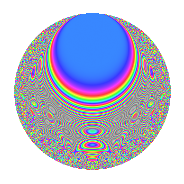Properties

 Label 6031.2.a.cLevel 6031 Weight 2 Character orbit 6031.a Self dual Yes Analytic conductor 48.158 Analytic rank 1 Dimension 110 CM No

Related objects

Newspace parameters

 Level: $$N$$ = $$6031 = 37 \cdot 163$$ Weight: $$k$$ = $$2$$ Character orbit: $$[\chi]$$ = 6031.a (trivial)

Newform invariants

 Self dual: Yes Analytic conductor: $$48.157777459$$ Analytic rank: $$1$$ Dimension: $$110$$ Fricke sign: $$1$$ Sato-Tate group: $\mathrm{SU}(2)$

$q$-expansion

The dimension is sufficiently large that we do not compute an algebraic $$q$$-expansion, but we have computed the trace expansion.

 $$\operatorname{Tr}(f)(q) =$$ $$110q$$ $$\mathstrut -\mathstrut 9q^{2}$$ $$\mathstrut +\mathstrut 97q^{4}$$ $$\mathstrut -\mathstrut 26q^{5}$$ $$\mathstrut -\mathstrut 26q^{6}$$ $$\mathstrut -\mathstrut 4q^{7}$$ $$\mathstrut -\mathstrut 27q^{8}$$ $$\mathstrut +\mathstrut 62q^{9}$$ $$\mathstrut +\mathstrut O(q^{10})$$ $$\operatorname{Tr}(f)(q) =$$ $$110q$$ $$\mathstrut -\mathstrut 9q^{2}$$ $$\mathstrut +\mathstrut 97q^{4}$$ $$\mathstrut -\mathstrut 26q^{5}$$ $$\mathstrut -\mathstrut 26q^{6}$$ $$\mathstrut -\mathstrut 4q^{7}$$ $$\mathstrut -\mathstrut 27q^{8}$$ $$\mathstrut +\mathstrut 62q^{9}$$ $$\mathstrut -\mathstrut 17q^{10}$$ $$\mathstrut -\mathstrut 9q^{11}$$ $$\mathstrut -\mathstrut 21q^{13}$$ $$\mathstrut -\mathstrut 29q^{14}$$ $$\mathstrut -\mathstrut 23q^{15}$$ $$\mathstrut +\mathstrut 79q^{16}$$ $$\mathstrut -\mathstrut 76q^{17}$$ $$\mathstrut -\mathstrut 31q^{18}$$ $$\mathstrut -\mathstrut 27q^{19}$$ $$\mathstrut -\mathstrut 67q^{20}$$ $$\mathstrut -\mathstrut 30q^{21}$$ $$\mathstrut -\mathstrut 28q^{22}$$ $$\mathstrut -\mathstrut 32q^{23}$$ $$\mathstrut -\mathstrut 63q^{24}$$ $$\mathstrut +\mathstrut 66q^{25}$$ $$\mathstrut -\mathstrut 55q^{26}$$ $$\mathstrut -\mathstrut 4q^{28}$$ $$\mathstrut -\mathstrut 81q^{29}$$ $$\mathstrut -\mathstrut 48q^{30}$$ $$\mathstrut -\mathstrut 30q^{31}$$ $$\mathstrut -\mathstrut 73q^{32}$$ $$\mathstrut -\mathstrut 53q^{33}$$ $$\mathstrut -\mathstrut 23q^{34}$$ $$\mathstrut -\mathstrut 78q^{35}$$ $$\mathstrut +\mathstrut 7q^{36}$$ $$\mathstrut -\mathstrut 110q^{37}$$ $$\mathstrut -\mathstrut 50q^{38}$$ $$\mathstrut -\mathstrut 64q^{39}$$ $$\mathstrut -\mathstrut 37q^{40}$$ $$\mathstrut -\mathstrut 123q^{41}$$ $$\mathstrut -\mathstrut 63q^{42}$$ $$\mathstrut -\mathstrut 40q^{43}$$ $$\mathstrut -\mathstrut 31q^{44}$$ $$\mathstrut -\mathstrut 73q^{45}$$ $$\mathstrut +\mathstrut 16q^{46}$$ $$\mathstrut -\mathstrut 37q^{47}$$ $$\mathstrut -\mathstrut 29q^{48}$$ $$\mathstrut +\mathstrut 46q^{49}$$ $$\mathstrut -\mathstrut 58q^{50}$$ $$\mathstrut -\mathstrut 73q^{51}$$ $$\mathstrut -\mathstrut 39q^{52}$$ $$\mathstrut -\mathstrut 16q^{53}$$ $$\mathstrut -\mathstrut 53q^{54}$$ $$\mathstrut -\mathstrut 59q^{55}$$ $$\mathstrut -\mathstrut 113q^{56}$$ $$\mathstrut -\mathstrut 39q^{57}$$ $$\mathstrut +\mathstrut 11q^{58}$$ $$\mathstrut -\mathstrut 93q^{59}$$ $$\mathstrut -\mathstrut 18q^{60}$$ $$\mathstrut -\mathstrut 66q^{61}$$ $$\mathstrut -\mathstrut 40q^{62}$$ $$\mathstrut -\mathstrut 21q^{63}$$ $$\mathstrut +\mathstrut 23q^{64}$$ $$\mathstrut -\mathstrut 92q^{65}$$ $$\mathstrut -\mathstrut 31q^{66}$$ $$\mathstrut +\mathstrut q^{67}$$ $$\mathstrut -\mathstrut 121q^{68}$$ $$\mathstrut -\mathstrut 80q^{69}$$ $$\mathstrut -\mathstrut 3q^{70}$$ $$\mathstrut -\mathstrut 75q^{71}$$ $$\mathstrut -\mathstrut 114q^{72}$$ $$\mathstrut -\mathstrut 39q^{73}$$ $$\mathstrut +\mathstrut 9q^{74}$$ $$\mathstrut -\mathstrut 25q^{75}$$ $$\mathstrut -\mathstrut 58q^{76}$$ $$\mathstrut -\mathstrut 31q^{77}$$ $$\mathstrut +\mathstrut 68q^{78}$$ $$\mathstrut -\mathstrut 36q^{79}$$ $$\mathstrut -\mathstrut 82q^{80}$$ $$\mathstrut -\mathstrut 50q^{81}$$ $$\mathstrut -\mathstrut 18q^{82}$$ $$\mathstrut -\mathstrut 57q^{83}$$ $$\mathstrut -\mathstrut 9q^{84}$$ $$\mathstrut -\mathstrut 14q^{85}$$ $$\mathstrut -\mathstrut 58q^{86}$$ $$\mathstrut -\mathstrut 58q^{87}$$ $$\mathstrut -\mathstrut 15q^{88}$$ $$\mathstrut -\mathstrut 181q^{89}$$ $$\mathstrut +\mathstrut 8q^{90}$$ $$\mathstrut -\mathstrut 55q^{91}$$ $$\mathstrut -\mathstrut 116q^{92}$$ $$\mathstrut -\mathstrut 86q^{93}$$ $$\mathstrut -\mathstrut 39q^{94}$$ $$\mathstrut -\mathstrut 70q^{95}$$ $$\mathstrut -\mathstrut 127q^{96}$$ $$\mathstrut -\mathstrut 91q^{97}$$ $$\mathstrut -\mathstrut 19q^{98}$$ $$\mathstrut -\mathstrut 21q^{99}$$ $$\mathstrut +\mathstrut O(q^{100})$$

Embeddings

For each embedding $$\iota_m$$ of the coefficient field, the values $$\iota_m(a_n)$$ are shown below.

For more information on an embedded modular form you can click on its label.

Label $$a_{2}$$ $$a_{3}$$ $$a_{4}$$ $$a_{5}$$ $$a_{6}$$ $$a_{7}$$ $$a_{8}$$ $$a_{9}$$ $$a_{10}$$
1.1 −2.78685 −0.498757 5.76655 1.84399 1.38996 −1.25608 −10.4968 −2.75124 −5.13894
1.2 −2.77072 1.69880 5.67692 −3.04963 −4.70691 3.28914 −10.1877 −0.114069 8.44968
1.3 −2.69148 −2.62726 5.24405 0.394241 7.07121 −0.479189 −8.73128 3.90249 −1.06109
1.4 −2.67187 1.06249 5.13890 3.00541 −2.83884 2.91292 −8.38673 −1.87111 −8.03007
1.5 −2.64482 −1.59347 4.99505 −2.42826 4.21443 4.09769 −7.92136 −0.460856 6.42231
1.6 −2.64313 2.88413 4.98613 −1.94351 −7.62312 −0.878417 −7.89274 5.31819 5.13695
1.7 −2.55998 2.69028 4.55348 −2.87781 −6.88705 3.57411 −6.53686 4.23759 7.36714
1.8 −2.51631 1.27091 4.33182 1.33976 −3.19800 0.944830 −5.86759 −1.38479 −3.37125
1.9 −2.48517 3.11043 4.17608 3.13519 −7.72995 −2.09644 −5.40793 6.67478 −7.79149
1.10 −2.45577 −2.50707 4.03080 −3.71982 6.15679 −2.33961 −4.98718 3.28541 9.13502
1.11 −2.41585 0.542785 3.83634 −2.85515 −1.31129 −2.66009 −4.43632 −2.70538 6.89763
1.12 −2.35892 −1.34501 3.56450 0.355993 3.17276 −3.31316 −3.69052 −1.19096 −0.839759
1.13 −2.33551 0.518713 3.45460 −1.17306 −1.21146 −2.50606 −3.39724 −2.73094 2.73970
1.14 −2.29692 −1.19284 3.27585 2.94374 2.73987 2.63830 −2.93052 −1.57712 −6.76154
1.15 −2.27127 −0.567339 3.15866 −3.83448 1.28858 2.52768 −2.63164 −2.67813 8.70914
1.16 −2.17795 2.23759 2.74348 1.66421 −4.87337 −3.06154 −1.61926 2.00681 −3.62457
1.17 −2.13628 −0.861354 2.56370 1.50403 1.84010 −3.07386 −1.20423 −2.25807 −3.21303
1.18 −2.12748 −2.55539 2.52618 2.02951 5.43655 −1.20737 −1.11945 3.53003 −4.31775
1.19 −2.05303 −2.17913 2.21492 2.99569 4.47382 4.05870 −0.441239 1.74862 −6.15023
1.20 −1.98962 1.97988 1.95861 0.230568 −3.93921 −0.245933 0.0823553 0.919909 −0.458744
See next 80 embeddings (of 110 total)
 $$n$$: e.g. 2-40 or 990-1000 Embeddings: e.g. 1-3 or 1.110 Significant digits: Format: Complex embeddings Normalized embeddings Satake parameters Satake angles

Inner twists

This newform does not have CM; other inner twists have not been computed.

Atkin-Lehner signs

$$p$$ Sign
$$37$$ $$1$$
$$163$$ $$1$$

Hecke kernels

This newform can be constructed as the kernel of the linear operator $$T_{2}^{110} + \cdots$$ acting on $$S_{2}^{\mathrm{new}}(\Gamma_0(6031))$$.